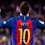# A probe to the sun

For the purpose of collescting data related to the space near the sun ,a probe is to be prepared that would be sending solar data back to the earth as it rushes towards it's demise.The design plan for our probe is that we will release it from our spacecraft in such a way that it has zero velocity relative to the sun.the probe has a mass $200$ kg and no rocket engine ,so it simply falls into the sun from rest due to the gravitational force from the sun.All other gravitational forces on the probe from other planets are neglected (for simplyfication) We will model the probe as a system consisting of a single particle.The gravitational force from the sun acts as the interaction with the environment ,doing work on thr probe .The result of this work is the increasing kinetic energy of the probe as it falls towards the sun .Thus situation is suitable for application of work energy theorem.

The initial separation of the probe and the sun is the radius of earth's orbit , $1.50\times 10^{11}$ m .The final separation is the radius of the sun $7\times 10^8$.The amount of work done by the gravitational force of the sun on the probe can be found using

$\displaystyle\int_{r_i}^{r_f}$$F_r$ $dr$

As the force is in radial direction , further

$W=\displaystyle\int_{r_i}^{r_f}$$F_r$$dr$$= -\displaystyle\int_{r_i}^{r_f}$$\dfrac{G\times{M_{sun}}\times{m_{probe}}}{r^2}=$$G\times{M_{sun}}$$\times{m_{probe}}\dfrac{r_i-r_f}{r_f\times{r_i}}$

Where G is univerasl gravitational constant = $6.7\times{10^{-11}}$N$m^2$/$kg^2$

mass of sun = $2.8\times {10^30}$,using $W=\triangle {K}$ , final speed can be calculated.

This is part of the set A probe to SunNote by Parth Lohomi
6 years, 3 months ago

This discussion board is a place to discuss our Daily Challenges and the math and science related to those challenges. Explanations are more than just a solution — they should explain the steps and thinking strategies that you used to obtain the solution. Comments should further the discussion of math and science.

When posting on Brilliant:

• Use the emojis to react to an explanation, whether you're congratulating a job well done , or just really confused .
• Ask specific questions about the challenge or the steps in somebody's explanation. Well-posed questions can add a lot to the discussion, but posting "I don't understand!" doesn't help anyone.
• Try to contribute something new to the discussion, whether it is an extension, generalization or other idea related to the challenge.
• Stay on topic — we're all here to learn more about math and science, not to hear about your favorite get-rich-quick scheme or current world events.

MarkdownAppears as
*italics* or _italics_ italics
**bold** or __bold__ bold
- bulleted- list
• bulleted
• list
1. numbered2. list
1. numbered
2. list
Note: you must add a full line of space before and after lists for them to show up correctly
paragraph 1paragraph 2

paragraph 1

paragraph 2

[example link](https://brilliant.org)example link
> This is a quote
This is a quote
    # I indented these lines
# 4 spaces, and now they show
# up as a code block.

print "hello world"
# I indented these lines
# 4 spaces, and now they show
# up as a code block.

print "hello world"
MathAppears as
Remember to wrap math in $$ ... $$ or $ ... $ to ensure proper formatting.
2 \times 3 $2 \times 3$
2^{34} $2^{34}$
a_{i-1} $a_{i-1}$
\frac{2}{3} $\frac{2}{3}$
\sqrt{2} $\sqrt{2}$
\sum_{i=1}^3 $\sum_{i=1}^3$
\sin \theta $\sin \theta$
\boxed{123} $\boxed{123}$

## Comments

Sort by:

Top Newest

try the problems here

- 6 years, 3 months ago

Log in to reply

There is some problem in the values given. Please rectify the problem asap

- 5 years, 9 months ago

Log in to reply

The whole set is problematic. Suggestion is, escape this Set now. I've Reported this set to the Brilliant Physics. As soon as its reviewed and corrected, I will let you know. Please drop your E-mail address.

- 5 years, 7 months ago

Log in to reply

rg51096@gmail.com

- 5 years, 5 months ago

Log in to reply

OK. You have an E-mail to check.

- 5 years, 4 months ago

Log in to reply

The answer to your question " A probe to sun-3 " comes out to be 16564.26877 m/s. But the answer given is 17000. Why? Though that's close but isn't the exact answer 16564 m/s. Please correct me if I am wrong somewhere.

- 5 years, 7 months ago

Log in to reply

The whole set is problematic. Suggestion is, escape this Set now. I've Reported this set to the Brilliant Physics. As soon as its reviewed and corrected, I will let you know. Please drop your E-mail address.

- 5 years, 7 months ago

Log in to reply

×

Problem Loading...

Note Loading...

Set Loading...